• 维空间与二维空间转换参数求解方法，王广兴 ，何薇，本文提出一种基准转换参数求解的新方法——椭球重合法（包括一般椭球重合法附加固定参数的椭球重合法），并且讨论了该方法方法
• 分别编写两个类Point2D，Point3D来表示二维空间和三维空间的点，使之满足下列要求： (1) Point2D有两个整型成员变量x, y (分别为二维空间的X,Y方向坐标)，Point2D的构造方法要实现对其成员变量x, y的初始化...
 分别编写两个类Point2D，Point3D来表示二维空间和三维空间的点，使之满足下列要求：
(1) Point2D有两个整型成员变量x, y (分别为二维空间的X,Y方向坐标)，Point2D的构造方法要实现对其成员变量x, y的初始化。
(2)Point2D有一个void型成员方法offset(int a, int b)，它可以实现Point2D的平移。
(3)Point3D是Point2D的直接子类，它有有三个整型成员变量x,y,z (分别为三维空间的X,Y,Z方向坐标)，Point3D有两个构造方法：Point3D(int x,int y,int z)和Point3D(Point2D p,int z)，两者均可实现对Point3D的成员变量x, y,z的初始化。
(4)Point3D有一个void型成员方法offset(int a, int b,int c)，该方法可以实现Point3D的平移。
(5)在一个测试类中的主函数main()中实例化两个Point2D的对象p2d1，p2d2，打印出它们之间的距离，再实例化两个Point3D的对象p3d1，p3d2，打印出他们之间的距离。
2D与3D既是独立又是继承关系.
主要用到:1.数学函数求两点之间的距离2.super的用法3.类的继承4.对象作函数参数
以下通过代码具体分析
import java.lang.Math; class Point2D{ protected int x,y;　　　　　　　　//保护类成员主要用于继承 Point2D(){ } Point2D(int x,int y){　　　　　　　//有参构造方法 this.x = x; this.y = y; } public int getX(){ return x; } public int getY(){　　　　　　　　//方便在其他类中使用该类中的私有成员变量 return y; } public void offset(int a,int b){    　　//偏移后的横纵坐标值 this.x += a; this.y += b; } public void show(){　　　　　　　　//show横纵坐标 System.out.println("坐标为：["+x+ "," +y +"]"); } } class Point3D extends Point2D{　　//类的继承 private int z;　　　　　　　　　　　//第三点x Point3D(){ } Point3D(int x,int y,int z){　　　　　　//有参构造方法三点分别初始化 super(2,3);　　　　　　　　　　　　//调用父类的有两个参数的构造方法 this.z= z; } public int getZ(){ return z; } Point3D(Point2D p,int z){　　　　　//有参构造方法,以对象作形参.用p对象调用get方法以初始化继承的私有成员变量 super(p.getX(),p.getY()); this.z=z; } public void offset(int a,int b,int c){　　//3D的偏移量 super.offset(a,b);　　　　　　　　　//方法同上,调用父类的偏移方法 z=z+c; } public void show(){　　　　　　　　//show3D坐标值 System.out.println("坐标为：["+x+ "," +y + "," + z +"]"); } } public class Test3_1 { public static void main(String[] args){ Point2D p2d1=new Point2D(2,3); Point2D p2d2=new Point2D(3,4);　　　　　//初始化2D两点p2d1,p2d2 double retDistance2D=distance2D(p2d1,p2d2); System.out.println(retDistance2D);　　　　//输出2D两点之间距离 Point3D p3d1=new Point3D(2,3,4); Point3D p3d2=new Point3D(3,4,5); double retDistance3D=distance3D(p3d1,p3d2); System.out.println(retDistance3D);　　　　//同上面的2D } public static double distance2D(Point2D p2d1,Point2D p2d2){//对象作形参 double distance; double distanceX; double distanceY; distanceX=Math.pow((p2d1.getX()-p2d2.getX()),2);//(x1-x2)2=X distanceY=Math.pow((p2d1.getY()-p2d2.getY()),2);//(y1-y2)2=Y distance=Math.sqrt(distanceY+distanceX);//X+Y再开方 return distance;   } public static double distance3D(Point3D p3d1,Point3D p3d2){//同2D方法一致 double distance; double distanceX; double distanceY; double distanceZ; distanceX=Math.pow((p3d1.getX()-p3d2.getX()),2); distanceY=Math.pow((p3d1.getY()-p3d2.getY()),2); distanceZ=Math.pow((p3d1.getZ()-p3d2.getZ()),2); distance=Math.sqrt(distanceY+distanceX+distanceZ); return distance;   } } 
展开全文• matlab在二维平面和三维空间中绘制圆形 二维平面绘制圆形 方法1： x = 10;y = 10;%圆心 r = 5; %半径 rectangle('Position',[x-r,y-r,2*r,2*r],'Curvature',[1,1],... 'FaceColor',[0.5 0.5 0.5],'EdgeColor',[0.5 ...
matlab在二维平面和三维空间中绘制圆形
二维平面绘制圆形
方法1：
x = 10;y = 10;%圆心
r = 5; %半径
rectangle('Position',[x-r,y-r,2*r,2*r],'Curvature',[1,1],...
'FaceColor',[0.5 0.5 0.5],'EdgeColor',[0.5 0.5 0.5],'linewidth',1,'LineStyle','-')
axis([0 100 0 100])
axis square
set(gca,'XTick',0:10:100);
set(gca,'YTick',0:10:100);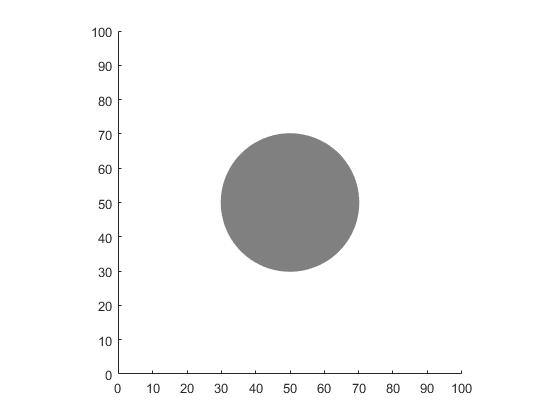方法2：
x = 30;  % 圆心
y = 40; % 圆心
r = 15; 半径
theta=0:0.01:2*pi ; % 圆滑性设置
Circle1=x+r*cos(theta);
Circle2=y+r*sin(theta);
plot(Circle1,Circle2,'Color','k','linewidth',1);
axis([0 100 0 100])
axis square
set(gca,'XTick',0:10:100);
set(gca,'YTick',0:10:100);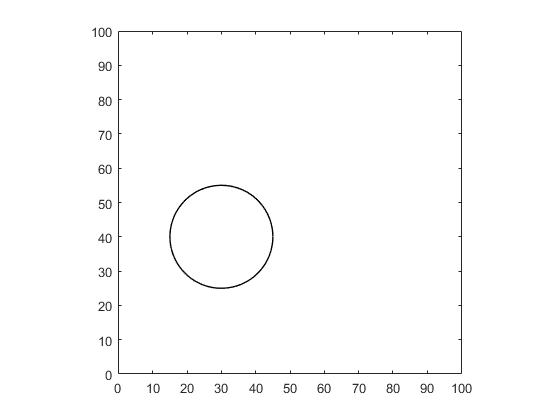三维空间绘制圆形
h = 5; % 高度
r = 20;  %半径
pos = [20,70]; % 圆心位置
t=0:0.001:(2*pi);  % 圆滑性设置
t=[t,0];
plot3(pos(1)+r*sin(t),pos(2)+r*cos(t), h*ones(size(t)))
axis([0 100 0 100])
axis square
set(gca,'XTick',0:10:100);
set(gca,'YTick',0:10:100);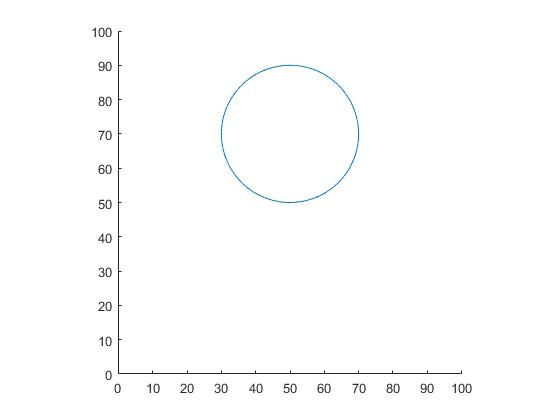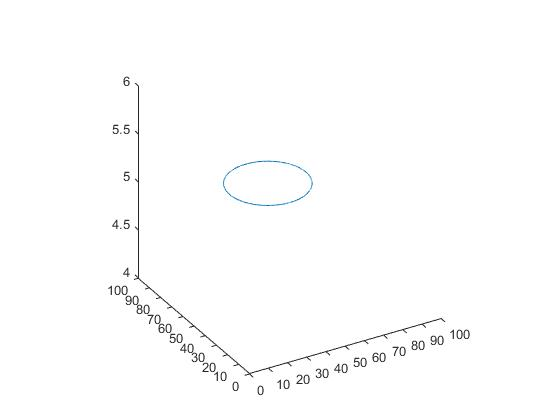展开全文matlab 圆形
• 从真实二维图像的集合合成三维空间的虚拟二维图像.zip
• 分别编写两个类Point2D，Point3D来表示二维空间和三维空间的点，使之满足下列要求原题分析代码 原题 分别编写两个类Point2D，Point3D来表示二维空间和三维空间的点，使之满足下列要求： Point2D有两个整型成员变量x...


分别编写两个类Point2D，Point3D来表示二维空间和三维空间的点，使之满足下列要求
原题分析代码

原题
分别编写两个类Point2D，Point3D来表示二维空间和三维空间的点，使之满足下列要求：
Point2D有两个整型成员变量x, y (分别为二维空间的X,Y方向坐标)，Point2D的构造方法要实现对其成员变量x, y的初始化。Point2D有一个void型成员方法offset(int a, int b)，它可以实现Point2D的平移。Point3D是Point2D的直接子类，它有有三个整型成员变量x,y,z (分别为三维空间的X,Y,Z方向坐标)，Point3D有两个构造方法：Point3D(int x, int y, int z)和Point3D(Point2D p, int z)，两者均可实现对Point3D的成员变量x, y, z的初始化。Point3D有一个void型成员方法offset(int a, int b, int c)，该方法可以实现Point3D的平移。在Point3D中的主函数main()中实例化两个Point2D的对象p2d1，p2d2，打印出它们之间的距离，再实例化两个Point2D的对象p3d1，p3d2，打印出他们之间的距离。
分析
这个题是对java中继承相关知识的应用，将其完整的写下来有助于增强我们对代码的熟练度。 具体要求题目计较明确，就是写两个类，然后再在主函数中运行，题目要求是在Point3D中的主函数main()中实例化相关对象，这里我想单独写一个主函数，感觉整体看起来会舒服一点，当然，你完全可以将单独出来的合并到一起。
代码
package Point;

public class Point2D {
protected int x;
protected int y;

public Point2D() {
}

public Point2D(int x, int y) {
this.x = x;
this.y = y;
}

public int getX() {
return x;
}

public void setX(int x) {
this.x = x;
}

public int getY() {
return y;
}

public void setY(int y) {
this.y = y;
}

public void offset(int a, int b) {
x += a;
y += b;
}

}


package Point;

public class Point3D extends Point2D {
private int z;

Point3D() {
}

Point3D(int x, int y, int z) {
super(x, y);
this.z = z;
}

public void setZ(int z) {
this.z = z;
}

public int getZ() {
return z;
}

Point3D(Point2D p, int z) {
super(p.getX(), p.getY());
this.z = z;
}

public void offset(int a, int b, int c) {
super.offset(a, b);
z = z + c;
}

}


package Point;

import java.math.*;

public class PointTest {
public static void main(String[] args) {
Point2D p2d1 = new Point2D(0, 0);
Point2D p2d2 = new Point2D(1, 1);
double length2D = distance2D(p2d1, p2d2);
System.out.println("两点之间的距离是：" + length2D);
Point3D p3d1 = new Point3D(0, 0, 0);
Point3D p3d2 = new Point3D(1, 1, 1);
double length3D = distance3D(p3d1, p3d2);
System.out.println("三点之间的距离是：" + length3D);
}

public static double distance2D(Point2D p2d1, Point2D p2d2) {
double distance;
double x;
double y;
x = Math.pow((p2d1.getX() - p2d2.getX()), 2);
y = Math.pow((p2d1.getY() - p2d2.getY()), 2);
distance = Math.sqrt(y + x);
return distance;
}

public static double distance3D(Point3D p3d1, Point3D p3d2) {
double distance;
double x;
double y;
double z;
x = Math.pow((p3d1.getX() - p3d2.getX()), 2);
y = Math.pow((p3d1.getY() - p3d2.getY()), 2);
z = Math.pow((p3d1.getZ() - p3d2.getZ()), 2);
distance = Math.sqrt(y + x + z);
return distance;

}

}展开全文java
• 225从真实二维图像的集合合成三维空间的虚拟二维图像_new.pdf
• 想要实现的功能就是已知网格三维坐标，如何将转成在某个视角下的二维坐标。说白了就是如何将三维坐标用电脑呈现出来（因为电脑平面是二维的）。比如下面这些三维坐标点。 具体步骤主要分为三步骤： 1、选定观察点P...
前言
其实这篇文章讲的就是类似于MATLAB中的mesh函数的实现原理。想要实现的功能就是已知网格三维坐标，如何将转成在某个视角下的二维坐标。说白了就是如何将三维坐标用电脑呈现出来（因为电脑平面是二维的）。比如下面这些三维坐标点。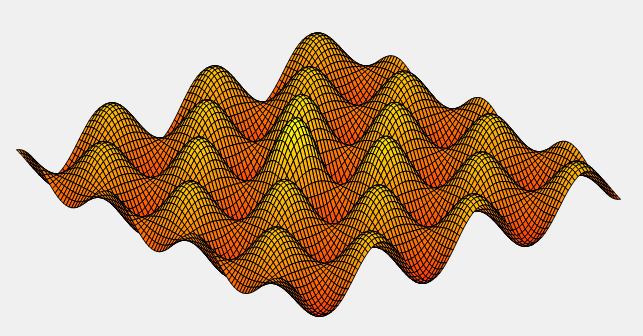具体步骤
主要分为三步骤：  1、选定观察点P（默认与x轴37度，与z轴30度，与原点距离（R）足够远）
2、通过空间坐标变换，将原来的空间坐标系转换为以观察点为原点,PO为z轴的空间坐标系。
3、通过投影将三维空间坐标映射到平行于xoy平面的画布上。
步骤一
选定观察点，如下图的观察点P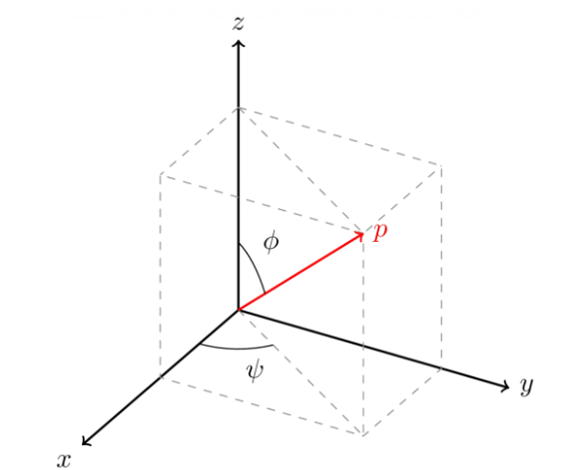步骤二
通过空间坐标变换，将原来的空间坐标系转换为以观察点为原点,PO为z轴的空间坐标系。  我们所说的空间坐标变换就是换个参考系，比如绕X,Y,Z轴旋转。可以感受一下（此三幅图，盗取的他人的，来源已不详，若给作者造成不便，还望与我联系）  绕X轴：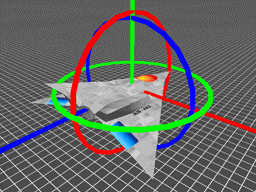绕Y轴：绕Z轴：以上是直观的显示，那我们如何求得变换后的坐标呢，这不，旋转矩阵派出用场了。
空间坐标变换——旋转矩阵（维基百科）  旋转可分为主动旋转与被动旋转。主动旋转是指将向量逆时针围绕旋转轴所做出的旋转。被动旋转是对坐标轴本身进行的逆时针旋转，它相当于主动旋转的逆操作。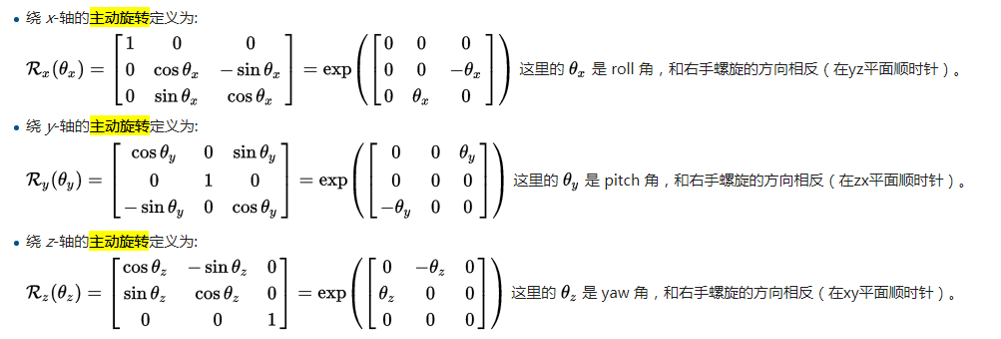所以要想实现第二步骤，则只需这两步：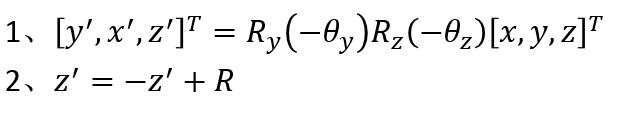步骤三
通过投影将三维空间坐标映射到平行于变换后参考系的xoy平面的画布上。  如下两图：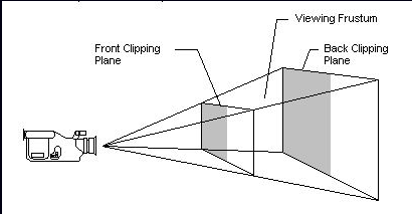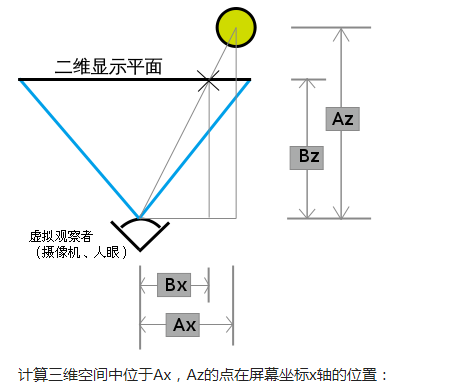先来解释第一幅图什么意思，第一幅图的意思是那个摄像机的位置就相当于观察点位置，那两平面左边的就是画布，右边代表的是经过任何一个待映射空间点Q且平行于xoy平面的平面。类似于从摄像机位置发出一条直线指向Q，与画布有一个交点R,我们最终要求的就是这个R在画布上的坐标。  第二幅图的意思就是如何求R在画布上的坐标，在这求x为例（求y类似）。途中绿点代表Q，图中的×代表R，我们会给定观察点与画布的距离。  所以R坐标求解如下图所示：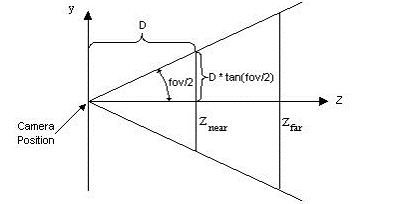大功告成！！！
展开全文• 就跟人没有翅膀一样，碰到吊在自己头顶的东西就只能绕远路通过二维的方法到达三维的地方，所以只要有工具，可以是翅膀，可以是深深固定地上的梯子，也可以是飞机，都可以从受限的二维空间达到三维空间。 而且这些...
• 关于camera calibration以后三维空间和二维图像之间的映射关系的讨论 多对一的映射 在三维空间内，任意曲面都可以通过摄像头的映射，投影到一个二维的图像之间。平行的三维空间的曲面重叠映射到同一个二维图像上...
• 二维图形 1.曲线图 Matlab作图是通过描点、连线来实现的，故在画一个曲线图形之前，必须先取得该图形上的一系列的点的坐标（即横坐标纵坐标），然后将该点集的坐标传给Matlab函数画图. 命令为： PLOT(X,Y,S) PLOT...matlab 数学建模
• 已知一段圆弧的起点、终点、弧度法向，求此段圆弧的参数方程参数区间...P(U) = O + R*Cos(U)*XDir + R*Sin(U)*YDir 这个问题主要应用于二维多段线中圆弧段的求解。在这里我先讲一下二维多段线的构造。 二维多段线圆弧
• 二维三维空间向量组的线性组合 向量的基本运算法则，构成了整个线性代数的基础。在物理学中，特别是求系统平衡时，经常碰到这类问题，给定若干矢量，这些矢量能与某特定矢量平衡吗？这就需要研究向量组的线性组合...线性代数 矩阵
• 最近突然想明白一件事儿。我把人与人，人与社会，人与自然的关系看成了一维、二维三维的关系。只要构成一个空间，就可以成为三个维度的关系。个体、团队、企业也是三维关系...部分人在一维和二维境界之间跳动，二...提高
• (1)Point2D有两个整型成员变量x, y (分别为二维空间的X,Y方向坐标)，Point2D的构造方法要实现对其成员变量x, y的初始化。 (2)Point2D有一个void型成员方法offset(int a, int b)，它可以实现Point2D的平移。 (3)...
• 平行的三维空间的曲面重叠映射到同一个二维图像上，通过透明重叠，形成一组实际的画面。平行的曲面上对应的无穷多个点映射到二维图像上一个相同的点，形成多对一的映射关系。 所以实际的三维空间在经过摄像头投影...
• 1．Viewport3D控件 查看详情》 Expression Blend中提供了Viewport3D控件，这是三维对象元素的上层容器，是相机对象元素Camera、三维图形呈现对象元素ModelVisual3D的集合。添加obj对象到【设计面板】后就自动建立...Viewport3D 3D
• 基于三维模型子空间监督学习法的二维人脸识别.pdf
• 最近在做项目的时候，遇到一个问题，这个问题的其中一个解决思路中用到了三维坐标转化成二维坐标。经过一段时间的研究，终于想出了解决方法。 预备知识： 空间平面表达式： Ax+By+Cz+d =0 空间直线表达式：（x-x0）...坐标系转化
• 行业分类-物理装置-一种使用二维激光雷达建立三维空间模型的系统及方法
• Matlab将二维图像三维重构源代码，把下面的代码写成m文件，运行就可以了。具体你要看到什么样的三维模型，你可以在此基础上修改。
• 同时在构建基于二维激光扫描仪（LMS291）的三维重建实验系统的基础上，研究分析了LMS291在平动转动两种不同工作模式下的工作特点并通过Matlab编写程序进行数据处理，利用MatlabFledermaus软件生成三维重建效果图...
• ## Matlab二维、三维作图

千次阅读 多人点赞 2019-01-23 08:19:05
二维作图命令 MATLAB 中最常用也是最基本二维作图命令为plot, plot函数针对向量或矩阵的列来绘制曲线的. 调用plot函数的常用格式有以下几种 • plot(x): 当x为一向量时, 以x元素的值为纵坐标, x的序号为横坐标值...Matlab
• 西南交通大学数学建模 Matlab绘图 Matlab 绘图 Matlab 绘图 手工作图 Matlab 绘图 Matlab ...其他相关命令 其他相关命令 同时绘制多个函数图像 空间三维作图 空间三维作图 空间三维作图 空间三维作图 二维作图机制 点 ?
• 常规状态下已入库的数据一般是二维的点面线数据，有时需要进行三维的显示：本篇我们来介绍如何对二维数据进行三维模型展示生成。 二维要素是否带高程Z判断 查看手中的数据是否带有高程Z值：二维面高程Z值的要素...GIS ARCGIS pro...